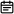# 53. 最大子序和2019-06-15

## 题目链接

https://leetcode-cn.com/problems/maximum-subarray/

## 题目描述

``````输入: [-2,1,-3,4,-1,2,1,-5,4],

## 解题方案

### 思路

• 标签：动态规划
• 这道题用动态规划的思路并不难解决，比较难的是后文提出的用分治法求解，但由于其不是最优解法，所以先不列出来
• 动态规划的是首先对数组进行遍历，当前最大连续子序列和为sum，结果为ans
• 如果`sum > 0`，则说明sum对结果有增益效果，则sum保留并加上当前遍历数字
• 如果`sum <= 0`，则说明sum对结果无增益效果，需要舍弃，则sum直接更新为当前遍历数字
• 每次比较 `sum``ans`的大小，将最大值置为`ans`，遍历结束返回结果
• 时间复杂度：O(n)

### 代码

• Java版本
``````class Solution {
public int maxSubArray(int[] nums) {
int ans = nums;
int sum = 0;
for(int num: nums) {
if(sum > 0) {
sum += num;
} else {
sum = num;
}
ans = Math.max(ans, sum);
}
return ans;
}
}``````
• JavaScript版本
``````/**
* @param {number[]} nums
* @return {number}
*/
var maxSubArray = function(nums) {
let ans = nums;
let sum = 0;
for(const num of nums) {
if(sum > 0) {
sum += num;
} else {
sum = num;
}
ans = Math.max(ans, sum);
}
return ans;
};``````

### 画解